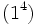# Hall-Senior classification of groups of order 16

This article describes the classification of the groups of order 16 by using the ideas of Hall-Senior genus and Hall-Senior family. This is one of many mutually similar classification approaches.

## Statement of classification

### Abelian groups

There is a unique Hall-Senior family, called$\Gamma_1$.

The nature and classification of the five abelian groups of order$p^4$ is the same for both the$p = 2$ and odd$p$ cases; the abelian groups are classified by the set of unordered integer partitions of the number 4. This follows from the structure theorem for finitely generated abelian groups. We do not discuss the classification of abelian groups further in this article, since it is common across all classification approaches.

Partition of 4 Corresponding abelian group (in general) Corresponding abelian group case$p = 2$ GAP ID (2nd part) case$p = 2$ Hall-Senior symbol Hall-Senior number
4 cyclic group of prime-fourth order cyclic group:Z16 1$(4)$ 5
3 + 1 direct product of cyclic group of prime-cube order and cyclic group of prime order direct product of Z8 and Z2 5$(31)$ 4
2 + 2 direct product of cyclic group of prime-square order and cyclic group of prime-square order direct product of Z4 and Z4 2$(2^2)$ 3
2 + 1 + 1 direct product of cyclic group of prime-square order and elementary abelian group of prime-square order direct product of Z4 and V4 10$(21^2)$ 2
1 + 1 + 1 + 1 elementary abelian group of prime-fourth order elementary abelian group:E16 14$(1^4)$ 1

### Class two groups

There is a unique Hall-Senior family, called$\Gamma_2$. This family is characterized as follows: the derived subgroup is isomorphic to cyclic group:Z2, the inner automorphism group is Klein four-group. If$P$ is the whole group, then the commutator map$P/Z(P) \times P/Z(P) \to [P,P]$ is fixed as the unique alternating bilinear map possible.

Here is a classification of the groups in this family:

Group Hall-Senior symbol Hall-Senior number Hall-Senior genus
direct product of D8 and Z2$16\Gamma_2a_1$ 6$16\Gamma_2a$
direct product of Q8 and Z2$16\Gamma_2a_2$ 7$16\Gamma_2a$
central product of D8 and Z4$16\Gamma_2b$ 8$16\Gamma_2b$
SmallGroup(16,3)$16\Gamma_2c_1$ 9$16\Gamma_2c$
nontrivial semidirect product of Z4 and Z4$16\Gamma_2c_2$ 10$16\Gamma_2c$
M16$16\Gamma_2d$ 11$16\Gamma_2d$

### Class three groups

There is a unique Hall-Senior family, called$\Gamma_3$. This family is characterized as follows: the derived subgroup is isomorphic to cyclic group:Z4, the inner automorphism group is dihedral group:D8, and the commutator map$P/Z(P) \times P/Z(P) \to [P,P]$ is fixed as the unique alternating bilinear map possible.

In fact, there is a unique Hall-Senior genus.

Here is a classification of the groups in this family:

Group Hall-Senior symbol Hall-Senior number Hall-Senior genus
dihedral group:D16$16\Gamma_3a_1$ 12$16\Gamma_3a$
semidihedral group:SD16$16\Gamma_3a_2$ 13$16\Gamma_3a$
generalized quaternion group:Q16$16\Gamma_3a_3$ 14$16\Gamma_3a$

## Proof of classification for nilpotency class two

### Proof of the uniqueness of the Hall-Senior family

'Given: A non-abelian group$P$ of order$16$, nilpotency class two.

To prove: If$Z(P)$ denotes the center of$P$, then$P/Z(P)$ is isomorphic to the Klein four-group, the derived subgroup$[P,P]$ is isomorphic to cyclic group:Z2, and the alternating bilinear map$P/Z(P) \times P/Z(P)$ to$[P,P]$ is defined as follows: the image of a pair of unequal non-identity elements is the non-identity element of$[P,P]$, and the image of equal elements and the image of a pair containing the identity element is the identity element.

Proof: Let$Z = Z(P)$.

Step no. Assertion/construction Facts used Given data used Previous steps used Explanation
1$Z$ is nontrivial. Fact (1)$P$ has order$2^4$ Fact-direct
2$Z$ cannot have order 16. --$P$ is non-abelian
3$Z$ cannot have order 8. Facts (2), (3), (4)$P$ is non-abelian, has order 16. [SHOW MORE]
4$Z$ has order either 2 or 4. Fact (4)$P$ has class two. Steps (1),(2),(3) [SHOW MORE]
5 If$Z$ has order 2, then$[P,P]$ has order 2.$P$ is non-abelian and has class two [SHOW MORE]
6 If$Z$ has order 2, then we get a contradiction PLACEHOLDER FOR INFORMATION TO BE FILLED IN: [SHOW MORE]
7 If$Z$ has order 4, then$P/Z$ is isomorphic to a Klein four-group,$[P,P]$ has order two, and the commutator map$P/Z \times P/Z \to [P,P]$ is as described. Facts (1), (4), (5), (6) [SHOW MORE]